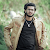# 18EE45 Electromagnetic Field Theory – FT Notes

[wptelegram-join-channel]

### 18EE45 Electromagnetic Field Theory – FT Notes

Here you can download the VTU CBCS 2018 Scheme notes, Question papers, and Study materials of 18EE45 Electromagnetic Field Theory.

University Name: Visvesvaraya Technological University (VTU), Belagavi

Branch Name: Electrical and Electronics Engineering – EEE

Semester: 4th Sem (2nd Year BE)

Subject Code and Subject Name: 18EE45 Electromagnetic Field Theory

Scheme of Examination: 2018 Scheme

Marks Distribution: 40 Marks for Continuous Internal Assessment and 60 Marks for Semester end examination

Important Concepts discussed:

Electric field intensity at a point due to an infinite sheet of charge. Electric field intensity at a point due to an infinite sheet of charge. Application of Gauss law, Maxwell’s First equation, Divergence theorem.

Energy and Potential, Conductors Dielectrics and Capacitance. Energy expended in moving a point charge in an electric field. Definition of Potential Difference and potential. The potential field of a point charge & system of charges.

Derivation of Poisson’s equation and Laplace’s equation. Uniqueness theorem, Examples of the solutions Laplace Equations and Poisson’s Equations.

Objectives, Introduction, Biot- savant law, Ampere’s circuital law, Curl, Stoke’s theorem, Magnetic flux and flux density, Scalar, and Vector magnetic potentials.

MAGNETIC FORCES, MATERIALS, AND DEVICES. Force on a Charged Particle. Force on a Current Element in a Magnetic Field. The force between Two Current Elements.

Materials in Magnetic Fields. Standard Inductance Configurations, etc.

Click the below link to download the 2018 Scheme VTU CBCS Notes of 18EE45 Electromagnetic Field Theory

M-1, M-2, M-3, M-4, and M-5

Click the below link to download the 2015 and 2017 Scheme VTU CBCS Notes of 15EE45 and 17EE45 Electromagnetic Field Theory

M-1, M-2, M-3, M-4 and M-5

1.